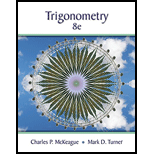Chapter 3.2, Problem 7PSTrigonometry (MindTap Course List)

8th Edition
Charles P. McKeague + 1 other
ISBN: 9781305652224

Solutions

Chapter
SectionTrigonometry (MindTap Course List)

8th Edition
Charles P. McKeague + 1 other
ISBN: 9781305652224
Textbook Problem

Find the radian measure of angle θ , if θ is a central angle in a circle of radius r , and θ cuts off an arc of length s . r = 12  inches , s = 3 π  inches

To determine

To find the radian measure of angle θ.

Explanation

Given information:

θ is the central angle in a circle of radius r=12 inches and is subtended by the arc of length s=3π inches.

.

By using the fact that magnitude of radians of subtended angle at the centre is equal to the ratio of arc length and radius of circle,

Still sussing out bartleby?

Check out a sample textbook solution.

See a sample solution

The Solution to Your Study Problems

Bartleby provides explanations to thousands of textbook problems written by our experts, many with advanced degrees!

Get Started

Find more solutions based on key concepts